Functionals: An Introduction to Variational Calculus by way of Variation of Parameters: Approximating the Geodesic

The introductory form of calculus of variations involves finding an unknown function, y = y(x),  which maximizes or minimizes a specified definite integral of y(x).

As an introductory problem, we might as a question such as, "of all paths that traverse a rolling landscape, which one is the shortest?"  The y(x) that minimizes the total length is called the geodesic.

The total length of a path over a fixed surface can be written as  an integral of the unknown y(x) (this is worked out in the accompanying lecture notes)
L[y(x)] =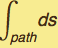=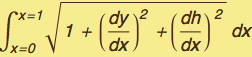Suppose that the "hill" has the shape h(x) =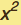.

Here, the "real" problem of finding the geodesic from the infinite set of all paths connecting y(x=0) = 0 to y(x=1) = 1  will be replaced by looking at all second-order polynomials: y(x) = a + bx + cand then minimizing over the (constrained) parameters. This will be compared with the exact calculation which we will work out below via calculus of variations.

We specify a landscape and an arbitrary quadratic path (a function of three parameters)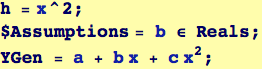Illustrate this surface and identify the starting and ending points.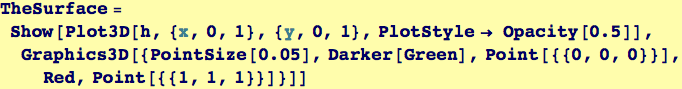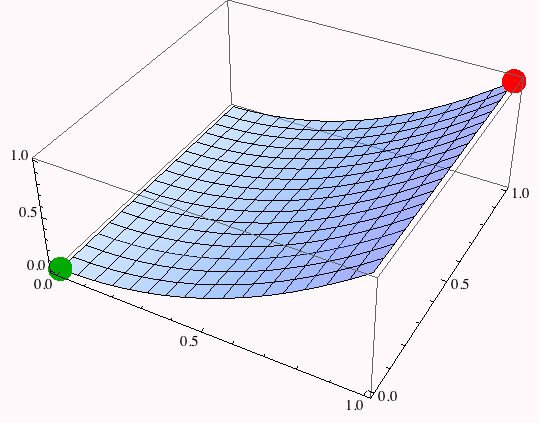The general path must satisfy the boundary conditions: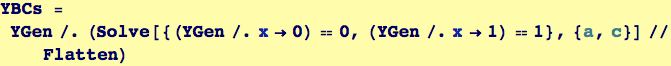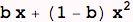There is one remaining free variable, it can be determined by minimizing the integral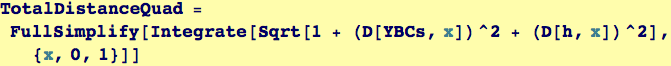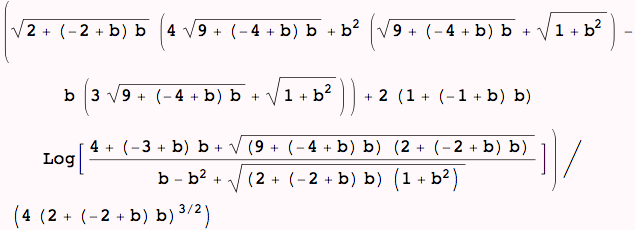To get an idea of how the path' s distance compares with its shape, we can manipulate the path and display that path' s length

Interactive Path Modification and Length Calculation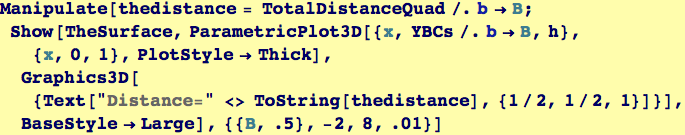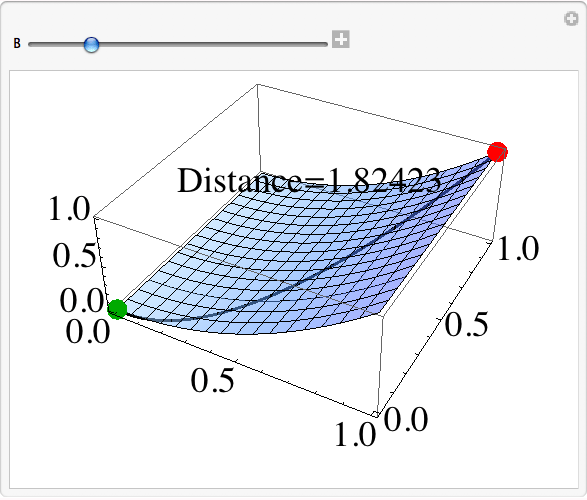Created by Wolfram Mathematica 6.0  (26 November 2007)